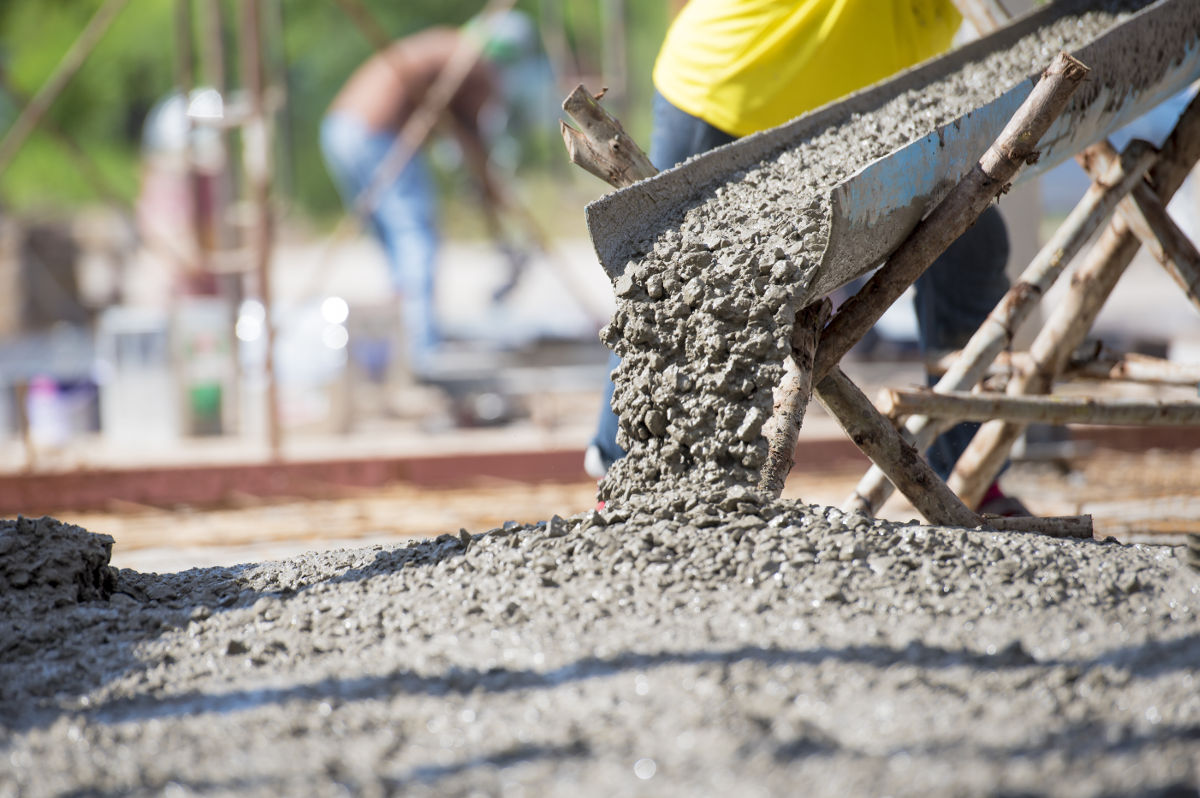# Concrete Weight Calculator

Find how much concrete weighs by entering the volume below. Use our concrete calculator to find the volume of concrete.

## Concrete Weight:

4,050
Pounds

Learn how we calculated this below

As an Amazon Associate, we may earn commissions from qualifying purchases from Amazon. Learn more

## How Much Does Concrete Weigh?

Concrete is a mixture of cement bonded with fine and coarse aggregates, including sand and crushed stone. This heavy material is used in many applications for building and construction.

A typical concrete mix weighs 150 lbs per cubic foot, 4,050 lbs per cubic yard, or 2,400 kg per cubic meter. The weight of concrete is determined by its density, which can vary based on the amount and type of aggregate, water, and air in the mix.

### How much does a yard of concrete weigh?

The average yard of concrete weighs 4,050 lbs. However, this can vary a little depending on different factors, such as the amount and type of aggregate that are added to the mixture.## How to Find the Weight of Concrete

You can calculate the weight of concrete in a few easy steps. Since the weight is determined by the density and volume, the first step is to calculate the density, then calculate the weight.

### Step One: Determine the Density of Concrete

Density is the measure of mass for a given volume and is defined by the formula:

density (ρ) = mass (m) ÷ volume (V)

The density of concrete is 3.15 g/cm3, while the density of aggregate will vary by the type of stone used. Most concrete will contain some amount of aggregate in the form of gravel or stone, which are added for strength. Some concrete mixtures use different levels and types of aggregate.

You will need to know the precise type of concrete and aggregate you are using for the most accurate weight. For instance, gravel has a much higher density than vermiculite. The density of a typical concrete mix is 2.4 g/cm3 or 150 lbs/ft3.

Different mixes of concrete will use more or less aggregate as well. For instance, the preferred concrete mix for a countertop will have less aggregate than the mix for a driveway or footing because the countertop does not need to be as strong, which will affect the density and, thus, the weight of the concrete.

Density of concrete in different units of measure
Volume Weight
1 in3 1.39 oz
1 ft3 150 lb
1 yd3 4050 lb
1 m3 2400 kg

### Step Two: Determine the Weight of Concrete

The density of concrete is determined by mass and volume. Since the density is known, the formula for mass can be changed by multiplying the density by the volume.

mass (m) = volume (V) × density (ρ)

For example, let’s find the weight of 25 cubic feet of concrete.

weight = 25 cu ft × 150 lb/cu ft
weight = 3,750 lbs

Tip: Use our cubic feet calculator to find the volume in feet.

For example, let’s find the weight of 3.5 cubic yards of concrete.

weight = 3.5 cu yd × 4,050 lb/cu yd
weight = 14,175 lbs

Tip: Use our cubic yards calculator to find the volume in yards.

You can use this method to calculate the weight of other materials or substances, such as the weight of water or even milk.